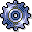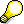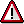Standard Distribution KeysUse

SAP provides the following standard distribution keys:

• Distribution key 0

Used to enter values manually for each period.

• Distribution key 1

Used to distribute the input value (annual plan value) equally across all plan periods.

• Distribution key 2

Used to distribute the input value (annual plan value) according to the last distribution key used.

• Distribution key 3

Interprets the input value as a percentage rate and multiplies it each period by the previous value.

• Distribution key 4

Used to distribute period values not equal to zero to the subsequent empty periods.

• Distribution key 5

Copies period values not equal to zero to the subsequent empty periods.The SAP R/3 System differentiates between true distribution keys and input help.

• If a plan value is distributed on periods using a true distribution key, the sum of all period values always equals the sum of the original plan values entered.
• If you use an input help, the original value entered may change.

The standard distribution keys 0, 3, 4, and 5 are input helps only. You change the entered parameters. The system does not save these distribution keys. This is so that new changes to the plan values can be avoided. Instead, it resets them to zero after use.

• Distribution key 6

Copies a given period value to subsequent periods.This distribution key has no effect on the manually planned values, as it is used on the period level. The distribution keys are, however, available on the annual level.

• Distribution key 7

Used to distribute the input value (annual plan value) among the individual periods in line with the number of calendar days per period.

• Distribution key 11

Can only be used in Cost Center Accounting for planning activity-dependent costs or activity-dependent statistical key figures.

The input value (annual plan value) is distributed in line with the activity quantity planned on the cost center.The R/3 System uses only those activity quantities planned at the time of the distribution key’s use. If you change activity quantities later or copy plan values to another version, the R/3 System does not automatically carry out new distributions.